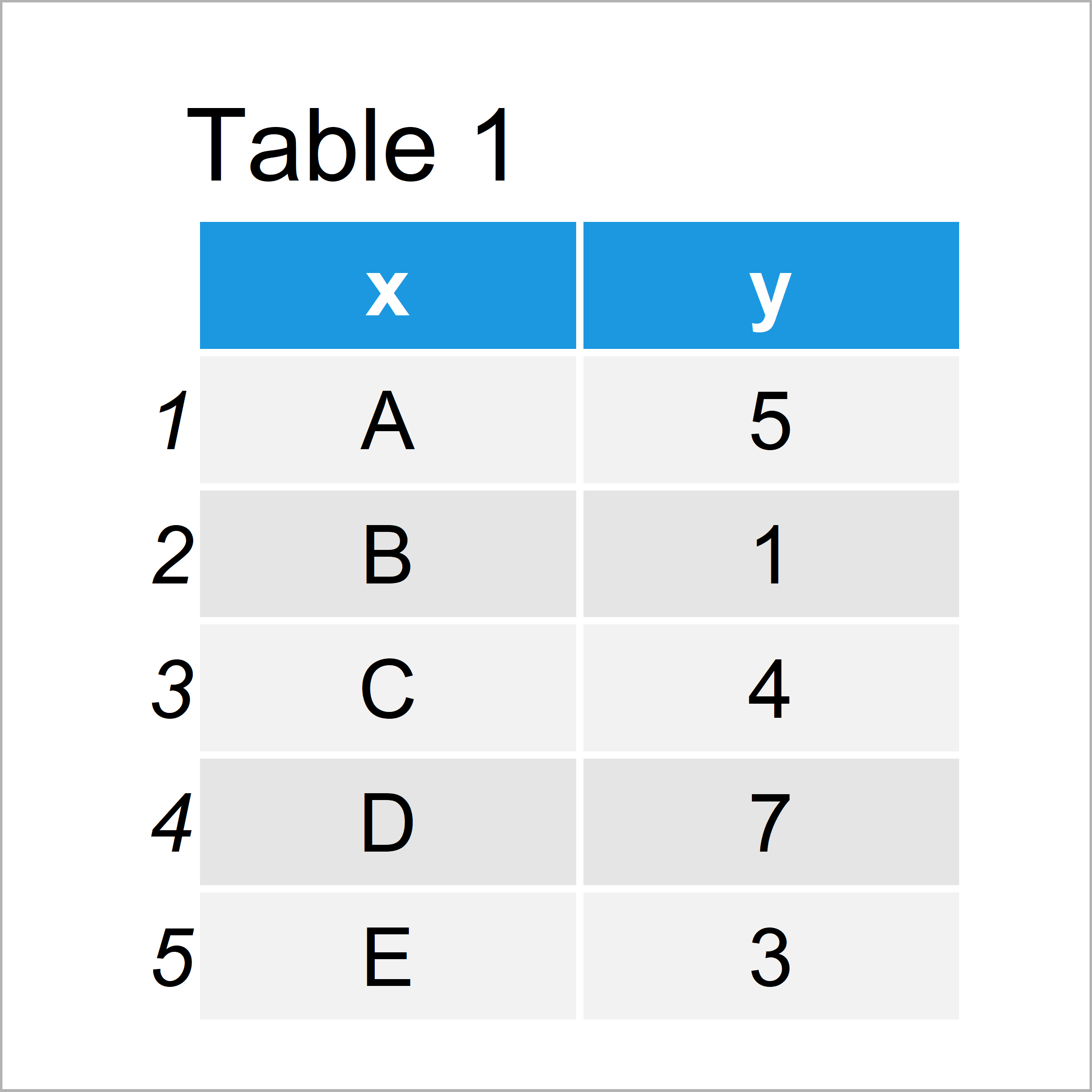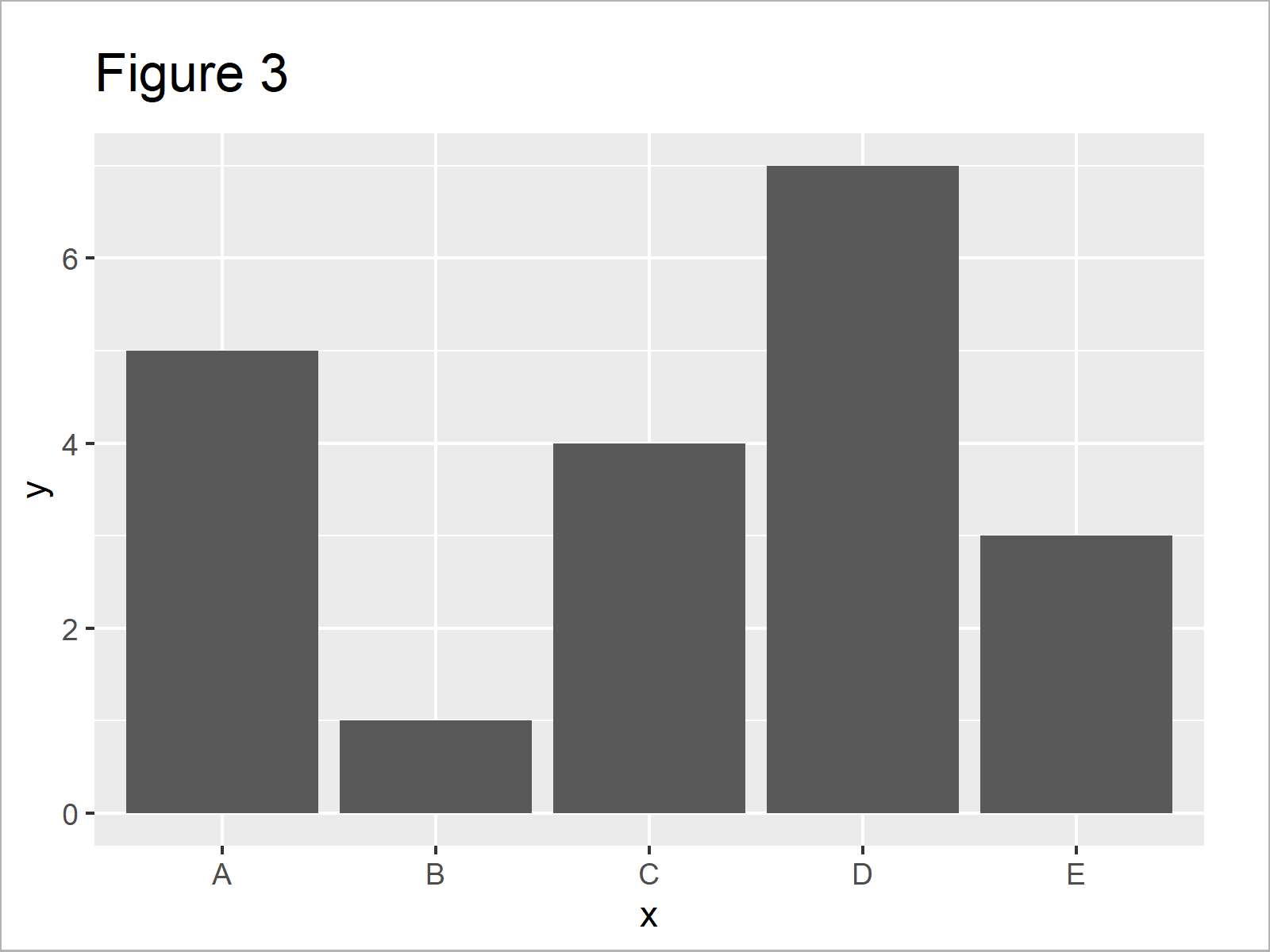# Increase Y-Axis Scale of Barplot in R (2 Examples)

This tutorial illustrates how to adjust the range of barchart ylim values in the R programming language.

The tutorial will consist of these contents:

## Example Data & Default Graph

The following data is used as basement for this R tutorial:

```data <- data.frame(x = LETTERS[1:5],    # Create example data
y = c(5, 1, 4, 7, 3))
data                                    # Print example data```Table 1 shows that our example data is constituted of five rows and two columns.

## Example 1: Increase Y-Axis Scale of Barchart Using Base R

The following syntax shows how to adjust the ylim range of a barplot created in Base R. Let’s first draw a barchart with default axis specifications:

`barplot(data\$y)                         # Base R barchart with default y-axis`The output of the previous syntax is shown in Figure 1: A bargraph with default y-axis values.

We can now increase the y-axis scale using the ylim argument:

```barplot(data\$y,                         # Base R barchart with manual y-axis
ylim = c(0, 15))```As shown in Figure 2, the previous syntax created a barplot with a y-axis ranging from 0 to 15.

## Example 2: Increase Y-Axis Scale of Barchart Using ggplot2 Package

In Example 2, I’ll illustrate how to change the y-axis scale of a ggplot2 barplot.

We first need to install and load the ggplot2 package:

```install.packages("ggplot2")             # Install ggplot2 package

Next, we can create and draw a ggplot2 barchart with default axes:

```ggp <- ggplot(data, aes(x, y)) +        # Create ggplot2 barchart with default y-axis
geom_bar(stat = "identity")
ggp                                     # Draw ggplot2 barchart with default y-axis```The output of the previous syntax is shown in Figure 3: A ggplot2 barplot with default axis ranges.

We can now use the ylim function to change our y-axis scale:

```ggp +                                   # Draw ggplot2 barchart with manual y-axis
ylim(0, 15)```As shown in Figure 4, we created a ggplot2 graphic with ylim ranging from 0 to 15with the previous R code.

## Video & Further Resources

In case you need more explanations on the R programming codes of this tutorial, you could have a look at the following video on my YouTube channel. I’m explaining the R code of this article in the video:

Please accept YouTube cookies to play this video. By accepting you will be accessing content from YouTube, a service provided by an external third party.If you accept this notice, your choice will be saved and the page will refresh.

Furthermore, I can recommend having a look at the related articles of this website:

To summarize: This article explained how to modify y-axis scales of barplots in the R programming language. Please let me know in the comments section, in case you have additional questions.

Subscribe to the Statistics Globe Newsletter